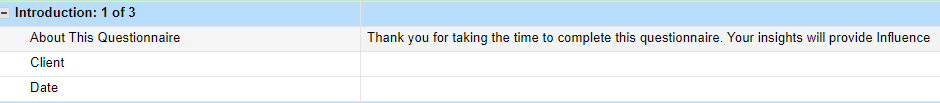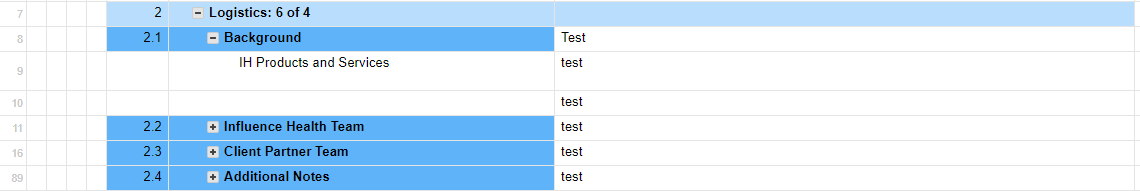# Tips for Showing Count of Answers Completed on a Smartsheet?

If I have a section with three rows underneath my heading, I'm able to easily use a formula to show the count of tasks completed/responded to out of my total number:

="Introduction: " + COUNT(Response4:Response6) + " of " + COUNT(CHILDREN())

However, if I have a section with multiple sub-sections, I'm struggling to use a formula to show this information. For example, my "Logistics" section has 4 sub-sections,  and the formula does not work for this. It will allow me to add more than my total number of children. See screenshot 2.

Does anyone have ideas on how to use a formula in this situation?• Hi Steven,

In short, it depends on how many sheets you need to implement this on.

If I'm understanding correctly, you'd need to have each subsection have a count(children) then sum(children) for the parent to get the answers.

Alternatively, a more complex formula leverages an if condition to say something along the lines of:

• if I am a child, throw 1 (or 0 if you wanna count unanswered)
• if I am a parent, sum the number of children (or answered children, etc)

This is done by creating a function that leverages ancestors and essentially asks "am I the lowest level".

Best,

Luke

## Help Article Resources

Want to practice working with formulas directly in Smartsheet?

Check out the Formula Handbook template!